Mathematical and Physical Journal
for High Schools
Issued by the MATFUND Foundation
 Already signed up? New to KöMaL?

# KöMaL Problems in Informatics, October 2015

Show/hide problems of signs:## Problems with sign 'I'

Deadline expired on November 10, 2015.

I. 382. ZUMA is a shooting game played on various boards. During the game the player should eliminate balls$\displaystyle -$having different colors and rolling along a line initially in one block$\displaystyle -$before any of the balls reach the edge of the board by shooting other balls at them. Create your program to simulate the game: the balls should roll along a straight line from left to right, and a shot is fired in each time unit. The detailed rules are as follows:

$\displaystyle \bullet$ initially all balls are located on the left of the board with no gaps between them;

$\displaystyle \bullet$ the first ball from the left is shifted to the right by one unit in each time unit;

$\displaystyle \bullet$ each ball is shifted whose neighbor has been shifted;

$\displaystyle \bullet$ a missile ball shot at the other balls reaches its destination after the shift described above took place, but still in the same time unit

$\displaystyle \circ$ if there is a ball at the impact position, then

$\displaystyle *$ if there is a block of balls adjacent to the impact position and having the same color as the missile color, then

- they disappear, that is, their positions become empty;

- if there are altogether at least 3 balls of the same color on the two sides of this newly created gap, then they also disappear, and this is repeated recursively;

$\displaystyle *$ otherwise the missile ball stays at the impact position, and the ball that was there is shifted to the right, and all balls to the right are shifted whose neighbor has been shifted;

$\displaystyle \circ$ if there is no ball at the impact position, then

$\displaystyle *$ if there is a ball in a neighboring position, then the missile ball stays at the impact position;

$\displaystyle *$ otherwise the missile ball disappears from the impact position.

The first line of the input file contains the length of the board $\displaystyle h$, the number of balls $\displaystyle p$ rolling on the board, and the number of balls $\displaystyle k$ shot during the game. The second line of the input contains $\displaystyle p$ characters (from the set A, ..., F) denoting the ball colors on the board and starting from the left. Then each of the next $\displaystyle k$ lines describes a ball--position pair: the first character denotes the missile ball color, and the second number is the impact position of this ball when it hits the board. The output shows the final state of the system after the last missile ball has been shot.

$\displaystyle \bullet$ If all balls were eliminated by the shots, then the first line of the output should be 0, and the second output line should be the number of the shot which first achieved this.

$\displaystyle \bullet$ If any of the balls reached the edge of the board, then the first output line should be -1, and the second output line should be the number of the shot after which this happened.

$\displaystyle \bullet$ If there is at least one ball on the board but none of them reached the edge of the board, then the first output line should be 1, and the second output line should contain $\displaystyle h$ characters denoting the ball colors still on the board from left to right, and with . denoting an empty position.

The following example shows 7 different and independent board positions, a shot, and the state of the board after this shot.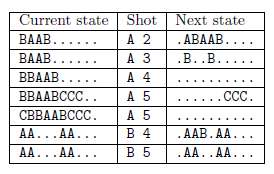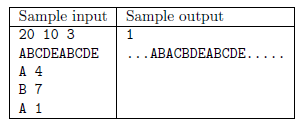The first and second command-line arguments to your program are the names of the input and output files, respectively.

The source code of your program$\displaystyle -$with a short documentation also describing your solution and specifying the name of the developer environment to use for compiling the source$\displaystyle -$should be submitted in a compressed file i382.zip.

(10 pont)

solution, statistics

I. 383. Data about some mining regions with license from the Hungarian authorities are available in the files telek.txt, banya.txt and nyersanyag.txt. The files are tabulator-separated, UTF-8 encoded text files with the first lines containing the field names.

Create a new database i383. First import the above data files into this database by using their original names. Upon importing, you should set the appropriate data formats and keys; the tables should not have any new fields.

Tables: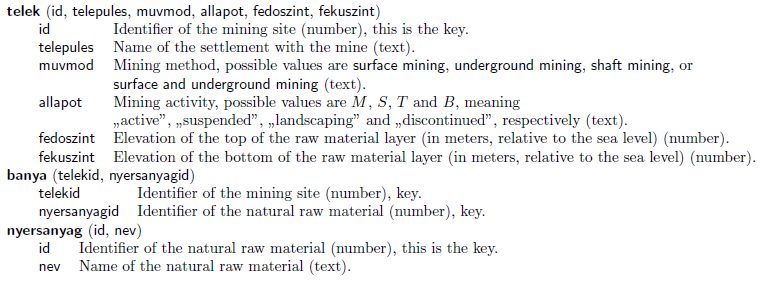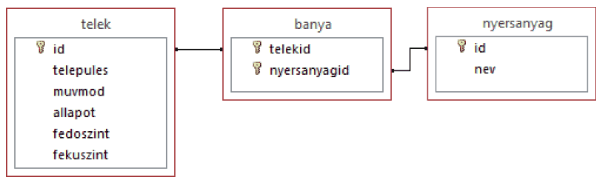You should solve the following tasks. For each query, only the requested values should appear and no other data. Your solutions should be saved by using the names specified in the parentheses.

1. By using a query, list all settlements where they discontinued underground mining. A settlement name cannot appear more than once in your list. (3bezart)

2. Which mining site has the thickest layer of coal? Display the settlement name and the layer thickness. (4sokszen)

3. For each rock mine determine, by using a query, what other raw materials are or were extracted. The list should not contain rock'' itself. (5kavics)

4. Determine the active mines in which there is extractable raw material between 400 and 500 meters above sea level. The list should contain the settlement of the mine and the name of the raw material. (6magas)

5. Various hydrocarbons$\displaystyle -$even in different state-of-matter forms$\displaystyle -$are likely to occur together. By using a query, list the settlement names where both oil and natural gas are extracted. The list should contain the settlement names and the number of mining sites with the above property. (7szenhidrogen)

6. To maintain the database, one needs to have data on the elevation of the top and bottom layers of the raw material. Create a report listing the sites with at least one missing piece of data. In the report you should highlight the settlement name, give the site identifiers for each mine, the name of the raw material extracted, and$\displaystyle -$if known$\displaystyle -$the elevation values for the topmost and bottommost layers. You should use a query or an auxiliary table to prepare the report. In your report, you should take into account the order of the fields, the title, and the way the field names are displayed in the sample. The format of your report can be different from that of the sample. (8hiany)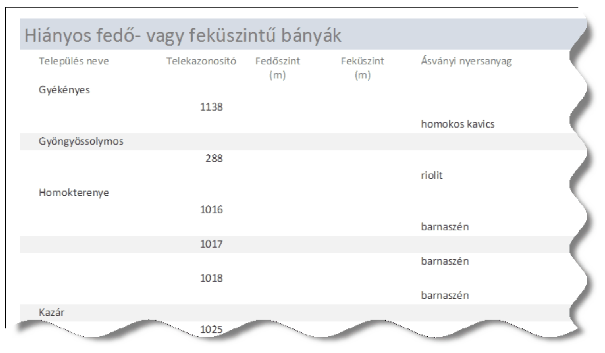7. Surface mining or surface and underground mining can vastly change the landscape. By using a query determine the number of settlements having any of these mining methods. (9tajrombolas)

The database with a short documentation (together with the name and version number of the database application) should be submitted in a compressed file i383.zip. Downloadable files: nyersanyag.txt, banya.txt, telek.txt (banyak.zip).

(10 pont)

solution, statistics

I. 384. Create an application to create and manipulate simple graphs (that is, graphs without loops or multiple edges). Your program should be able to handle and edit graphs with at most 20 vertices, and the user should be able to save and read these from a text file.

Upon clicking on an empty area in edit mode, a new vertex should be drawn. If the user clicks on two vertices, then the first vertex and the second vertex should become connected with an (undirected) edge if there was no edge between them, or the edge should be removed if there was already one between them.

Right-clicking with the mouse should have an analogous meaning: drawing or deleting a directed edge. The user should be able to move vertices with a drag-and-drop operation. Your program should number the vertices starting from 1. A double-click on a vertex should delete that vertex. If a vertex is removed, then all its edges should also be removed, and the numbers of all larger vertices should be decreased by one. Hence in the graph with $\displaystyle N$ vertices, the vertex numbers range from 1 to $\displaystyle N$. Vertices should be displayed as simple disks, with the current vertex numbers inside the disk. Edges should be drawn as line segments, and directed edges should have arrows. Your program can ignore certain graphical issues, for example, it is not considered as a problem if a disk partially covers an edge of a different vertex, or if two disks representing two vertices overlap. We can assume that the user is experienced, and tries to create a nice graph layout. Upon pressing key M, or choosing Save from the menu, your program should save the graph data into a text file graf.txt. The first line of the file should contain the number of vertices $\displaystyle N$ and edges $\displaystyle E$, then the width and height of the drawing area, separated by a space. The following $\displaystyle N$ lines contain the coordinates of the vertices obtained from the graphical input (pairs of integers separated by a space). The next $\displaystyle E$ lines should contain the edge data (again, pairs of integers separated by a space). Upon pressing key B, or choosing Open from the menu, your program should erase the current working area and open the file graf.txt for further editing.

In your solution you can use any programming language or developer environment that appears on our Rules of the contest'' page. We also accept web applications running on the client side.

The source code of your program$\displaystyle -$together with a short documentation also describing your solution and specifying the developer environment to compile your code$\displaystyle -$should be submitted in a compressed file i384.zip.

(10 pont)

solution, statistics## Problems with sign 'I/S'

Deadline expired on November 10, 2015.

I/S. 2. In this task you should determine the $\displaystyle k^\mathrm{th}$ permutation among all, lexicographically ordered permutations of length $\displaystyle n$ ($\displaystyle 1\le n\le 14$ és $\displaystyle 1\le k\le n!$).

A permutation of length $\displaystyle n$ can be thought of as a sequence of numbers $\displaystyle 1,2, \ldots, n$ in some given order. A permutation $\displaystyle p_1$ is said to precede another permutation $\displaystyle p_2$ in the lexicographic order, if the first different digit (read from the left) of $\displaystyle p_1$ is smaller than that of $\displaystyle p_2$. For example, the permutation $\displaystyle 2314$ for $\displaystyle n=4$ precedes the permutation $\displaystyle 2341$, so we write $\displaystyle 2314< 2341$.

Your program should read the values of $\displaystyle n$ and $\displaystyle k$ from the first line of the standard input, then write the corresponding permutation to the first and only line of the standard output.

 Sample input: Sample output: 4 2 1 2 4 3

Scoring and bounds. You can get 1 point for a brief and proper documentation clearly describing your solution. Nine further points can be obtained provided that your program solves any valid input within 1 second of running time.

The source code of your program without the .exe or any other auxiliary files generated by the compiler but with a short documentation$\displaystyle -$also describing which developer environment to use for compiling the source$\displaystyle -$should be submitted in a compressed file is2.zip.

(10 pont)

solution, statistics## Problems with sign 'S'

Deadline expired on November 10, 2015.

S. 101. We are given the map of a mountain range as a grid of $\displaystyle N\times M$ numbers containing the elevation data for each coordinate (every elevation is at most $\displaystyle 1 \,000\,000\,000$. We are also given some nice locations in the mountains. We would like to determine at least how difficult a trip is which starts from some nice location and visits every nice location. In other words, we aim to determine the smallest number $\displaystyle C$ with the following property: it is possible to start from any nice location and arrive at any other nice location by always proceeding from a square to one of its 4 neighbors with elevation difference at most $\displaystyle C$.

First your program should read the values of $\displaystyle N$ and $\displaystyle M$ ($\displaystyle 1\le N, M\le 800$, being the number of rows and columns of the map, respectively) from the first line of the standard input. Then read $\displaystyle M$ integers, representing elevation data, from each of the following $\displaystyle N$ lines. Next read again $\displaystyle M$ integers from each of the following $\displaystyle N$ lines: every integer is either 0 or 1 depending on whether the current location is nice or not, respectively. Your program should write the smallest possible appropriate $\displaystyle C$ in the first and only line of the standard output.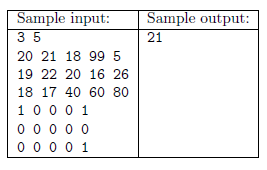Scoring and bounds. You can get 1 point for a brief and proper documentation clearly describing your solution. Nine further points can be obtained provided that your program solves any valid input within 1 second of running time.

The source code of your program without the .exe or any other auxiliary files generated by the compiler but with a short documentation$\displaystyle -$also describing which developer environment to use for compiling the source$\displaystyle -$should be submitted in a compressed file s101.zip.

(10 pont)

solution, statistics

\$Var(Body)